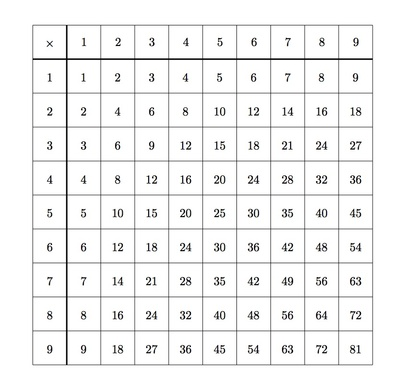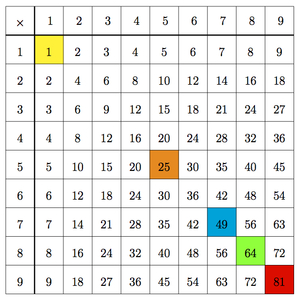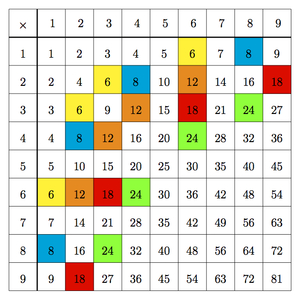# Numbers in a Multiplication Table

Alignments to Content Standards: 4.OA.B

The table below shows all the products of pairs of numbers between 1 and 9.1. Which numbers appear only once in the table of products? Where are these numbers located in the table? Why?
2. Which numbers appear the most frequently in the table? Why?
3. Which numbers between 10 and 20 do not appear in the table? What do these numbers have in common?

## IM Commentary

The goal of this task is to encourage students to study the multiplication table, a familiar object, from a novel point of view. The table shows some, but not necessarily all, factorizations of different numbers. For example, 24 appears 4 times in the table: $$24 = 3 \times 8 = 8 \times 3 = 6 \times 4 = 4 \times 6.$$ For the 9 by 9 multiplication table shown, only the numbers 1 through 9 appear with all of their factorizations. Working through the table to see where different numbers appear, the students will have a good opportunity to observe the symmetry of the table which comes from the commutative property of multiplication: $a \times b = b \times a$.

The last part of this question gives an opportunity for the teacher to discuss prime numbers since the list of numbers the students produce will all be primes. This makes sense in the context because a prime number cannot be written as a product of two smaller whole numbers. The first non-prime number not appearing in the table is $22 = 2 \times 11$, the product of the smallest prime number with the smallest prime number bigger than 9.

The main standard for mathematical practice which aligns with this task is MP7, Look For and Make Use of Structure, since the goal of the task is to help students understand the multiplication table from the point of view of factorizations. If students work on this task in groups and share their insights, then it is also a good opportunity to engage in MP3, Construct Viable Arguments and Critique the Reasoning of Others.

## Solution

1. The numbers which only appear once in the table are 1, 25, 49, 64, and 81. These are all along the diagonal of the table. These squares are each shaded in different colors in the picture below:This makes sense because a number such as 48 = 6 $\times$ 8, not on the diagonal, also appears as 48 = 8 $\times$ 6. This is the commutative property of multiplication and several of these squares are shaded below:For a number on the diagonal like $49 = 7 \times 7$, the two factors are the same and so when we switch their order, we don't get a different entry in the table. Some products on the diagonal, such as 2 $\times$ 2 = 4, do also appear elsewhere because they have a different factorization (4 $\times$ 1 and 1 $\times$ 4 in this case). If we extended the table to include products of all numbers, then every number $n$ (including all perfect squares) would appear at least twice as $n\times1$ and $1\times n$.

2. Several numbers appear 4 times in the table: 6, 8, 12, 18, and 24. These are shown below:There are some numbers, such as 9, 16, and 36, which appear 3 times. The numbers which appear 4 times have two distinct factorizations into single digit numbers (up to the order of the factors): for example, $24 = 4 \times 6 = 3 \times 8$. If the table were continued further, other larger numbers such as 72 and 48 would appear many more than 4 times. The number 72 will eventually appear 12 times and the number 48 will appear 10 times.

3. We see that 10, 12, 14, 15, 16, 18, and 20 all appear in the table so the missing numbers between 10 and 20 are $$11, 13, 17, 19.$$ The multiplication table contains all products of single digit numbers. So a number not in the table can not be factored as a product of two single digit numbers. In the case of 11, 13, 17, and 19, this means that they do not factor at all, except as a product of 1 and themselves. So this list is the list of prime numbers between 10 and 20.